Reach Us+441414719275
Simulation of Propagation of Action Potentials in Cardiac Tissue with an Inhomogeneous Distribution of Extracellular Potassium | OMICS International
Journal of Computer Science & Systems Biology

Like us on:

All submissions of the EM system will be redirected to Online Manuscript Submission System. Authors are requested to submit articles directly to Online Manuscript Submission System of respective journal.

# Simulation of Propagation of Action Potentials in Cardiac Tissue with an Inhomogeneous Distribution of Extracellular Potassium

Sudarshan Dhungana1 and Piyush Kar2*

1Oakland University, Rochester, MI, USA

2University of Alberta, Edmonton, Alberta, Canada

*Corresponding Author:
Piyush Kar
University of Alberta
Tel: 514-7122130
E-mail: [email protected]

Received date: November 04, 2015; Accepted date: November 25, 2015; Published date: November 30, 2015

Citation: Dhungana S, Kar P (2015) Simulation of Propagation of Action Potentials in Cardiac Tissue with an Inhomogeneous Distribution of Extracellular Potassium. J Comput Sci Syst Biol 8:6 373-379. doi:10.4172/jcsb.1000212

Copyright: © 2015 Dhungana S, et al. This is an open-access article distributed under the terms of the Creative Commons Attribution License, which permits unrestricted use, distribution, and reproduction in any medium, provided the original author and source are credited.

Visit for more related articles at Journal of Computer Science & Systems Biology

#### Abstract

We report simulation of experimental data for heterogeneous distribution of extracellular potassium, by developing and applying a mathematical model formulated by incorporating a Luo-Rudy representation of the cell membrane current into the bidomain model of the cardiac tissue. Our model serves to examine propagation of extra cellular potassium from a region of low potassium (normal) to a region of high potassium (ischemic). Starting with computer simulation of unipolar stimulation of cardiac tissue, we reproduced results of the passive bidomain model, and also simulated the space-clamped Luo Rudy model. Finally, we combined the Luo-Rudy model with the bidomain model. The diastolic threshold for anodal stimulation first decreased and then increased with increasing potassium concentration ([K]0), reaching a minimum value at [K]0=12mM.

#### Keywords

Mathematical modeling; Luo-Rudy model; Bidomain model; Extracellular potassium; Cardiac tissue; Action potential

#### Introduction

Our mind works and our heart beats as long as there is propagation of action potentials in our body. Action potentials are generated by the inward and outward movements of numerous ions, such as Na+, K+, Ca2+, Cl- across the cell membrane. These action potentials travel in the form of waves through the various chambers of the heart. To fully understand the heart, a detailed knowledge of action potential propagation is essential.

In unhealthy (ischemic) tissue, the extracellular potassium concentration, [K]0, is elevated. High [K]0 is believed to predispose cardiac tissue to break excitation (Roth and Patel) , which in turn plays an important role in re-entry induction and defibrillation. Because fibrillation raises extracellular [K]0 levels, break excitation may play a more important role in defibrillation than is suggested by simulations and experiments using normal [K]0 values. In their 2003 paper, Roth and Patel theoretically simulated action potential data in a 1 cm2 tissue verifying that the action potential may fail to propagate in an ischemic region. The wave propagates for [K]0=2 to 12.5mM and at higher potassium concentrations the wave dies out.

Sidorov et al. studied propagation in ischemic tissue experimentally. Their optical mapping experiment in a rabbit heart using [K]0 values of 4, 6, 8, 10 and 12 mM indicated that spatial heterogeneity of the action potential duration (APD) restitution, created with regional elevation of [K+]0, can lead to action potential (AP) instability, 2:1 block, and reentry induction .

The goal of this research is to simulate Sidorov et al. experiment using a mathematical model by incorporating a Luo-Rudy representation of the cell membrane current with the bidomain model of the cardiac tissue, and to examine propagation of potassium from a normal to an ischemic region.

#### Methods

Luo rudy model

Hodgkin and Huxley published a landmark paper that paved the way for subsequent electrophysiological models. They successfully explained the propagation of action potentials in the giant squid axon by representing the membrane resistance as resulting from ion flow through three ion channels: sodium, potassium and leakage . Each pathway was accompanied by a battery that had a potential equal to the Nernst potential for that ion. Variable resistances signifying the various ion channels opening and closing were expressed in terms of their conductance. The probability of opening or closing of these ionic channels was governed by three gating parameters m, n and h following the first order kinematics.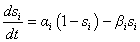(1)

In eq. (1), αi and βi represent the rate of opening and closing of the m, n, and h gates. These rate constants depended on the transmembrane voltage.

Hodgkin and Huxley wrote down the rate of change of membrane potential as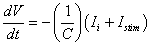(2)

Following the mathematical formulation of Hodgkin and Huxley, in 1991 Luo and Rudy developed a model of the ventricular cardiac action potential. They introduced and analyzed more ion channels then other contemporary physiological models. They replaced the three ionic current terms in Hodgkin and Huxley’s model with six other currents. They were a fast inward sodium Na+ current INa, a slow inward calcium Ca++ current ISi, a time dependent delayed rectifier potassium K+ current Ik, and time-independent potassium inwardly rectifying current Ik1, the plateau K+ current Ikp, and a time independent background current Ib. The membrane current depended on [K+]0 as a parameter in this model. Considering a membrane patch of area 1 cm2 with capacitance 1 μF/cm2 equation (2) can be rewritten as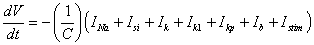(3)

All these ionic currents also depend on the gating variables, which are actually probabilistic values indicating the closing and opening of various ion channels. The time variation is given as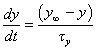(4)

where,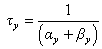(5)

and,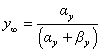(6)

Here, y can be any of the gating variables (m,h,….), τy is the time constant, and y is the steady state value of y. αy and βy are voltage dependent rate constants.

The fast inward sodium current in eq. (3) was described using the Ebihara-Johnson model  (Ebihara and Johnson) coupled with the slow inactivation gate (j) of the Beeler-Reuter model (Beeler and Reuter) ,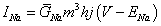(7)

where,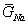is the ,…maximum conductance of the sodium channel (23 mS/cm2); m the activation parameter and h the inactivation parameter; and ENa is the reversal potential of sodium.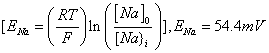(8)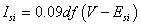(9)

where,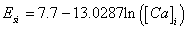(10)

and, d and f are two other gating parameters.

The delayed outward rectifier current Ik was based on a formulation proposed by Shibasaki and included a time–dependent activation gate X, a time independent (fast) inactivation gate Xi capturing the inward rectification properties of the delayed rectifier current, and a conductance modulated by extracellular K+ concentration .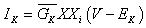(11)

where,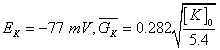(12)

The formulation of Ik1 was based on patch clamp data of Sakmann and Trube as well as other data from single ventricular myocytes .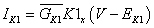(13)

where,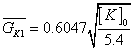(14)

The voltage-dependent and time independent plateau current that activates at depolarized plateau potentials was identified by Yue and Marban ,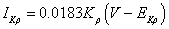(15)

Where, Ekp= Ek1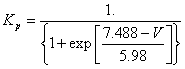(16)

The background current is formulated as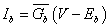(17)

where,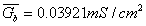,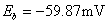Then the total time independent current is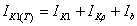(18)

Finally the rate of calcium uptake is given by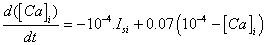(19)

complete listing of all the governing equations, parameters and their corresponding values can be found in Appendix.

Bidomain model

The two or three dimensional cable model, more commonly known as the bidomain model, describes the interaction of electric current with cardiac tissue. It was developed in the late 1970’s (Muler and Markin, Miller and Geselowitz, Tung) [9-11] and was applied to more problems in cardiac electrophysiology in the 1980s (Plonsey and Barr, Barr and Plonsey, Roth and Wikswo) [12-14].

Here we will solve the coupled parabolic and elliptic equations using a finite difference technique (Roth) and discuss some consequences of unipolar stimulation of cardiac tissue . The first analysis of this kind of work was done by Sepulveda et al. . The starting point here is to solve the set of two coupled partial differential equations governing the transmembrane potential, Vm, and extracellular potential, Ve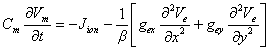(20)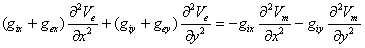(21)

To solve the parabolic type of equation, we use the simple Euler method. The idea here is to solve eq. (20) for the new value of Vm, and then solve the elliptic eq. (21) for the new value of Ve using the new value for Vm as a source term, by over-relaxation.

By, rearranging, we get,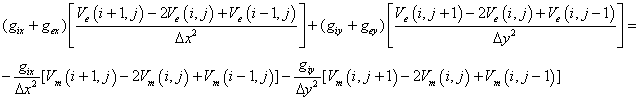(22)

In eq. (22), let,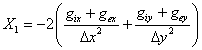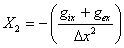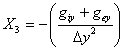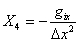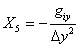So, we can write an expression for extracellular potential, Ve in terms of Vm, as follows: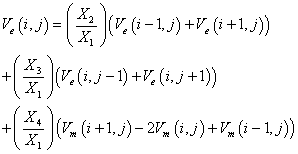#### Results and Discussion

By developing a code using the FORTRAN software, we simulated the distribution of extracellular potassium by using the bidomain model with a LR action potential. Sepulveda et al. used a passive bidomain model to study unipolar stimulation of cardiac tissue . They found that near a unipolar cathode, the tissue is strongly depolarized under the cathode, and more weakly hyperpolarized in two regions near the cathode along the fiber direction. Figure 1 shows our model generated transmembrane potential produced around the cathode, located at the origin.

Figure 1: Transmembrane potential induced in a 2D sheet of tissue by unipolar extracellular cathode, located at the origin. The fibers are along the X axis.

In presence of the cathode the tissue is depolarized (positive transmembrane potential) in a dogbone shape. However, about 2 mm from the cathode, in the direction along the fibers, is a region of hyperpolarization (negative), called a “virtual anode”. In Figure 1, only one quarter of the tissue is shown, so two virtual anodes exist one on each side of the cathode. The values in the plots are given in volts. Figure 2 shows anodic stimulation, which gives rise to two virtual cathodes on either side of the origin. Figure 3 shows the calculated transmembrane potential during unipolar cathodal stimulation of an anisotropic twodimensional sheet of passive cardiac tissue in a color plot.

Figure 2: Transmembrane potential induced in a 2D sheet of tissue by unipolar extracellular anode located at the origin.

Figure 3: Color plot of cathodal stimulation in a two dimensional sheet of cardiac tissue. The electrode is at the center of the tissue, and the fibers are horizontal.

A space clamped calculation of the LR action potential was performed in Luo and Rudy’s original paper (Luo and Rudy) . Figure 4 shows simulated action potentials for different extracellular concentrations of potassium.

Figure 4: Action potentials for [K]0=7, 5.4, 4, and 3 mM, calculated using the space-clamped Luo-Rudy model.

As [K]0 decreases, action potential duration increases and resting potential becomes more negative. Figure 5 shows the time course of IK1(T), IK, and Isi during the action potential. A peak in IK1(T) is observed during repolarization. For [K]0=7mM the peak is almost 3 μA/cm2 but for [K]0=3mM it is less than 2 μA/cm2. This large difference in IK1(T) brings about a faster rate of repolarization at higher [K]0. Also the peak of IK1(T) occurs earlier (at more positive membrane potential), reflecting an increase of the reversal potential EK1.

Furthermore, Figures 5-7 indicates that during repolarization the total time independent potassium current depends strongly on [K]0, while the time–dependent potassium current (IK) and slow inward current exhibit only weak dependence on [K]0.

The 1991 Luo-Rudy Phase I model did not describe intracellular Ca2+ cycling, sarcolemmal Na+-Ca2+ exchange, Na+-K+ pumping and temporal variation of intracellular ion concentrations . Luo and Rudy produced another model called Luo-Rudy phase II model, which is one of the most popular cardiac ventricular cell models even today (Luo and Rudy) . However, because focus is made on extracellular potassium concentration and not on intracellular calcium dynamics, it is not relevant here.

Our next analysis is made by combining the bidomain model with an active Luo-Rudy membrane, which bears similarity with simulations performed previously by Roth . Figure 8 shows stimulation threshold as a function of [K]0.. The threshold initially decreases as [K]0 increases, but then rises abruptly for [K]0 above 12mM. Figure 9 shows the resting potential as a function of potassium ion concentration. The resting potential follows approximately the potassium Nernst potential, with a very negative value at low [K]0 and then rising to less negative values as [K]0 increases.

Figure 9: Resting potential as a function of extracellular potassium ion concentration. The straight line represents the potassium Nernst potential.

Having verified that the model gives results similar to previous calculations, we can now apply the model to simulating the experiments of Sidorov et al. . We can consider a tissue that has [K]0=4mM (normal) on the left half of the tissue, and [K]0=10mM (ischemic) on the right (Figure 10). The tissue sheet is 10 mm by 10 mm (100 × 100 nodes), with a space step in each direction of 0.1 mm.

Figure 10: Two-dimensional sheet of tissue with normal (left) and ischemic (right) regions. Following an extracellular stimulus of 20 mV along the left edge, applied for the time periods, shown in the table, an action AP wave spreads from normal to ischemic tissue.

We can stimulate the tissue by raising the extracellular potential along the left edge to 20 mV at time t=0. An action potential then propagates across the tissue from left to right. Plots of the transmembrane potential versus time at three locations are shown in Figures 11 and 12. Point A (x=-4.92 mm, y=-4.92 mm) is near the left edge of the tissue sheet in the region of normal [K]0, point B (x=-0.025, y=-0.025) is at the center (just on the boundary between normal and ischemic tissue), and point C (x=4.9 mm, y=4.9 mm) is near the right edge in the region of elevated [K]0.

Figure 11: Action potential wave. The 2nd stimulus produced no AP because the tissue is refractory. Time interval chosen to apply stimulus is below the diagram (small table).

Figure 12: The transmembrane potential when the second stimulus is applied in the interval 542< t ≤ 543 ms. It can be observed that both, the first and second action potential waves, pass through the normal and ischemic tissue.

The second stimulus was applied at various times, but never found a case where propagation was successful in the normal tissue but then failed to enter the ischemic tissue. Also attempt was made by applying more than two stimuli (up to five) with various timings, and varied the distribution of extracellular potassium so that in one case the left was 5.4mM and the right was 8mM (Figure 13), and in another case the left was 8mM and the right was 12mM (Figure 14). Nevertheless, after extensive simulations none of these simulations resulted in propagation on the left but then failure on the right.

Figure 13: AP wave with left region 5.4 mM and right at 8 mM. Time interval chosen, were: 100< t< 101; 520< t < 521; 920< t< 921; and 1720 < t< 7211; the left but then failure on the right.

Figure 14: AP wave with left region 8 mM and right at 12 mM but for the same time intervals and stimulus voltage as in Figure 13.

We validated our calculations by combining both models by verifying previous calculations, in that we reproduced results reported by Sepulveda et al. , Luo-Rudy action potential (Luo and Rudy, 1991) and by Roth and Chen [17,19]. This exercise brought about the conclusion that resting potential rises with [K]0, which tends to make propagation easier (lower threshold) initially as [K]0 increases, but makes propagation harder (higher threshold) for very high [K]0 (Figures 8 and 9).

Finally the Sidorov et al. experiment was simulated. In their case, the wave front propagated from a region of [K]0=4mM to a region of [K]0=10mM in a Langendorff-perfused rabbit heart . They often found cases in which propagation was successful in the normal (4mM) tissue but then failed at the boundary between the normal and ischemic (10mM) tissue. Here this behavior was never observed. The reason appears to be the dependence of action potential duration on [K]0. In the Luo-Rudy model, the action potential duration decreases as [K]0 increases, so that the refractory period in the ischemic region is short. Any action potential that can propagate through the normal tissue (with a long refractory period) should have no problem also propagating through the ischemic tissue (with a short refractory period). Interestingly, in Sidorov et al. data, the action potential duration (and therefore the refractory period) appeared insensitive to extracellular potassium ion concentration. This appears to be the primary difference between our simulations (reported herein) and their experiments. Future simulations of Sidorov et al.’s data would need to include a model of the membrane kinetics that properly captures the [K]0 dependence of the action potential duration.

#### Conclusions

We simulated Sidorov’s experiment, in rabit heart, using a mathematical model, wherein we incorporated Luo Rudy’s representation of the cell membrane current with a bidomain model of the cardiac tissue.

In addition to matching experimental results by Sidorov, reliability of our model is also demonstrated by reproduction results of previously reported models that predicted dependence of extracellular potassium on action potential duration.

Using our model, we examined the propagation of potassium from low to high concentration and found that [K]0 is dependent on the action potential duration. Further research is necessary to refine our modelling approach by making it more inclusive of physiological parameters.

#### Acknowledgments

We thank Professor Bradley Roth (Oakland University) for his important suggestion and careful editing of the manuscript.

#### References

Appendix

Governing equations, parameters and their corresponding values for the Luo Rudy Model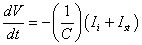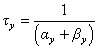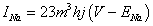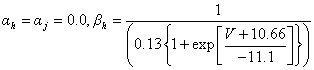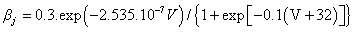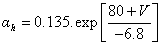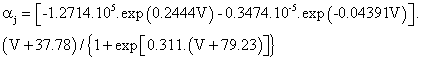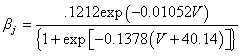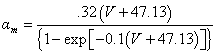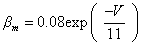Slow inward Current: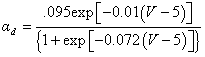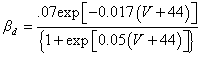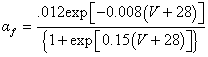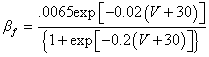Calcium uptake: d([Ca]i)/dt=-10-4.Isi+0.07(10-4-[Ca]i)

Expressions for outward currents are given below.

Time dependent Potassium Current: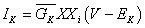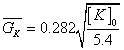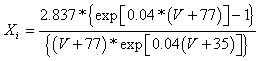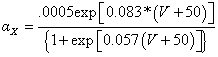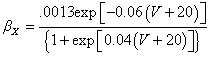Time- independent potassium current: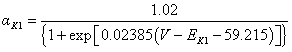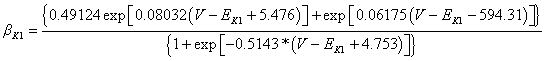Plateau Potassium current:Background current: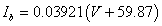Total time independent potassium current:Select your language of interest to view the total content in your interested language

### Article Usage

• Total views: 9181
• [From(publication date):
November-2015 - Jul 17, 2019]
• Breakdown by view type
• HTML page views : 9042Can't read the image? click here to refresh